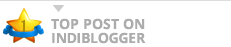# Accuracy upto how many decimals?32 + 42 = 52 9 + 16 = 25 (3/4)2 + (4/4)2 = (5/4)2 0.752 + 12 = 1.252 0.5625 + 1 = 1.5625 (3/5)2 + (4/5)2 = (5/5)2 0.62 + 0.82 = 12 0.36 + 0.64 = 1 ok Fellas, let’s notice a difference here. (3/3)^2 +(4/3)^2  = (5/3)^2 1^2 + 1.3^2 = … More Accuracy up

### PiyushGoel

blogs from GrNoida